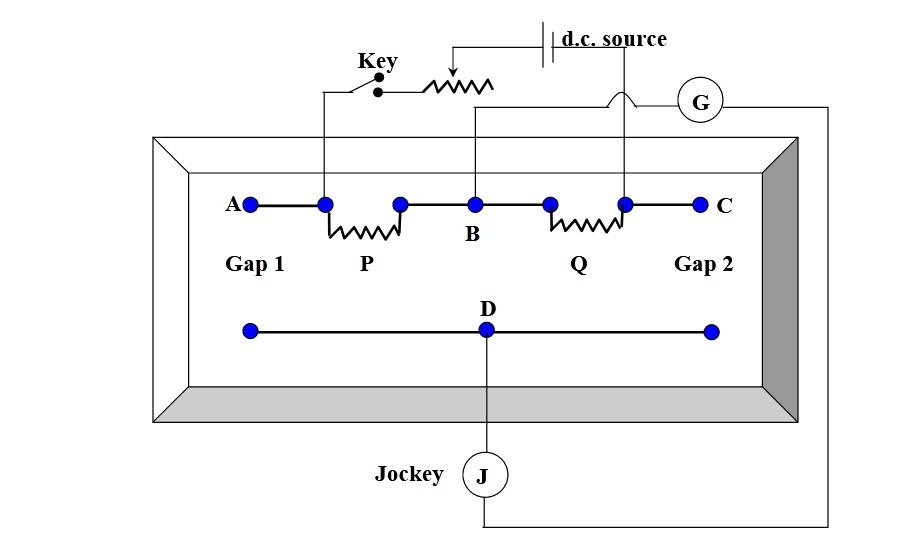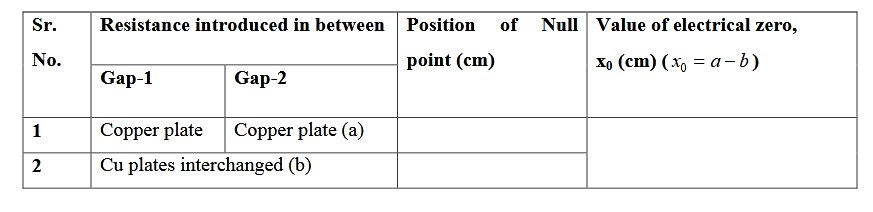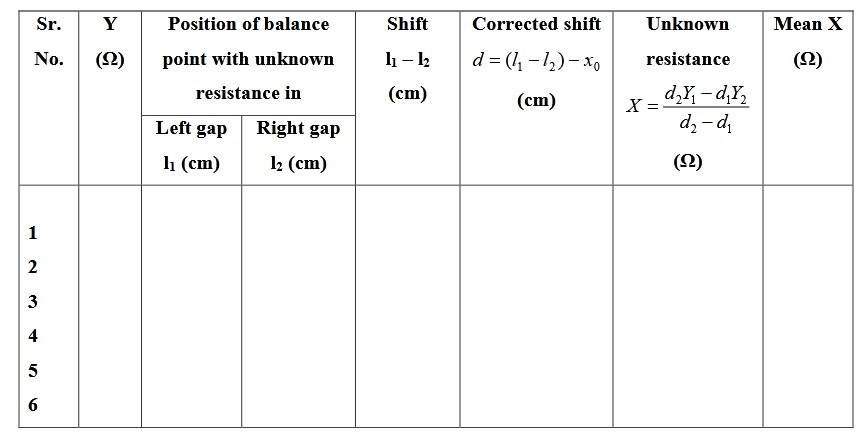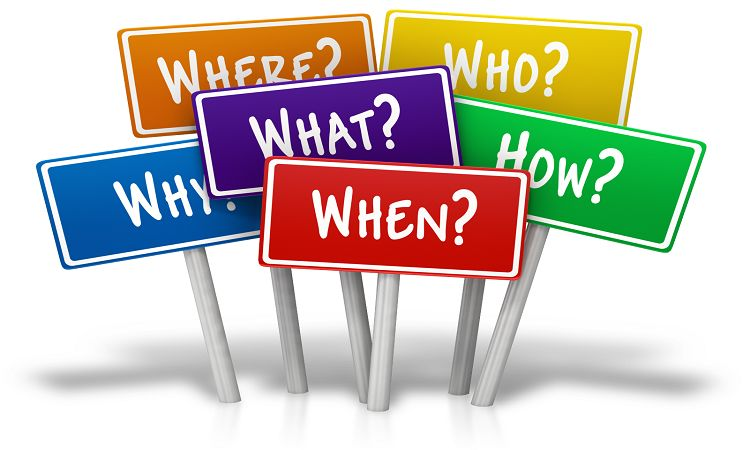# To find the low resistance by Carey Fosters Bridge Experiment Setup## Objective / Aim of the Experiment

To find the low resistance by Carey Fosters Bridge Experiment Setup

### Apparatus Required

Low Resistance
DC Power Supply (2V)
Carey Fosters Bridge Setup
Galvanometer
One way key

### Formula Used

X = (d2Y1 - d1Y2) / (d2-d1)

### Circuit Diagram of Carey Foster Bridge Experiment### Theory

Carey Fosters bridge is a modified Wheatstone bridge. Here a potentiometer wire MN is inserted between the R and S arms of the Wheatstone bridge as shown in Figure above. The ratio arms P & Q are made equal. Gap 1 carries a small resistance (known) and the Gap 2 (fourth arm) carries the unknown resistance. If ρ be the resistance per unit length of wire, r1 & r2 be the end resistances at M & N, MD = l1 is balancing length a shown in figure.

then P / Q = (X + l1ρ + r1) / [Y + (100-l1)ρ + r2] ............. (1)

When, X & Y are interchanged, the balance point shifts to a length 2, then,

then P / Q = (Y + l2ρ + r1) / [X + (100-l2)ρ + r2] ............. (2)

Comparing (1) & (2) and adding 1 to both sides, we get,
Y - X = (l1 - l2

Where X is unknown resistance, (l1 - l2) is shift in balance point when the positions of X & Y are interchanged. Let d1 & d2 are the shifts corresponding to resistances Y1 & Y2, then,

X - Y1 = d1ρ and X - Y2 = d2ρ, so, (X - Y1) / (X - Y2) = d1 / d2

X = (d2Y1 - d1Y2) / (d2-d1)

### Procedure

1. Make connection as shown in the figure.

2. Fix P = Q = 1 Ω throughout the experiment.

3. Keep X = Y = 0 by short circuiting by copper plates to get balance point.

4. Repeat step (iii) by interchanging the position of copper plates and determine x 0 .

5. Replace trip of gap 1 by unknown resistance X and gap 2 by known resistance Y. Find balance point for 0.1 Ω, and, then interchange X & Y, and, again find balance point.

6. Repeat this step for Y = 0.2, 0.3, 0.4, 0.5 Ω.

### Observations

(a) Determination of electrical zero:(a) Determination of unknown resistance:### Result

The value of unknown resistance is .............. Ω.

### Precautions

• All terminals should be tight.
• The connecting wires and the copper strip should be thoroughly cleaned with sand paper.
• The connection should be tight and the plugs of the resistance box should be given twist so that they are tight.
• The battery key should be taken out when the readings is not being taken in order to avoid heating and the wire.

### Carey Fosters Bridge Viva Questions and Answers

Question-1: What do you mean by the resistance of a conductor?
Answer-1: The ratio of the potential difference between the two ends of a conductor to the current flowing in it, is called the resistance of a conductor.

Question-2: On what factor does it depends?
Answer-2: Resistancve of a conductor is directly proportional yo its length(l), inversely proportional to the area of cross section (A). It also depends upon the nature of material and temperature of the conductor.

Question-3: What is its unit?
Answer-3: Unit if resistance is ohm.

Question-4: What is effect of temperature on resistance?
Answer-4: It increases with the increase in temperature.

Question-5: What is the effect of increasing the effective length of a Carey Fosters bridge wire?
Answer-5: It will increase the accuracy of the result because then percentage error in reading the position of the balance point is very much decreased.

Question-6: What is the minimum difference in resistance that you can measure with this bridge wire?
Answer-6: It is equal to the resistance of the one millimeter length of the bridge wire.

### Latest Articles### Spreading Knowledge Across the World

USA - United States of America  Canada  United Kingdom  Australia  New Zealand  South America  Brazil  Portugal  Netherland  South Africa  Ethiopia  Zambia  Singapore  Malaysia  India  China  UAE - Saudi Arabia  Qatar  Oman  Kuwait  Bahrain  Dubai  Israil  England  Scotland  Norway  Ireland  Denmark  France  Spain  Poland  and  many more....